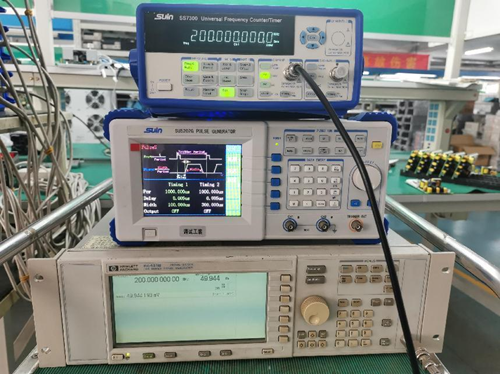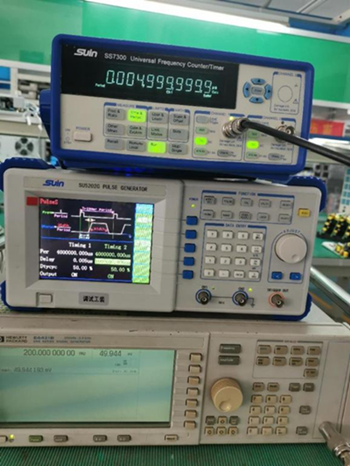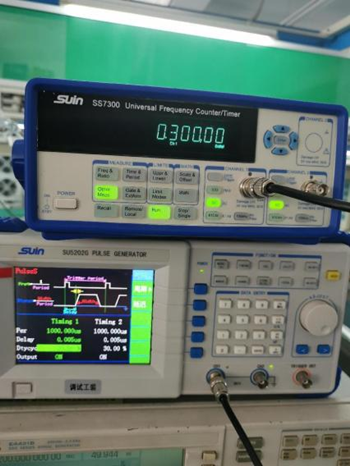News

# Brief Introduction of SS7000 Series Universal Frequency Counter/Timer/Analyzer

Sep. 26, 2022

What's a frequency counter? It is an electronic test instrument especially made for signal frequency measuring and is mainly composed of a time base circuit, input circuit, frequency display circuit and control circuit.

Along with the development of technique and R&D capability, the instruments made by China perform more and more excellently than that made overseas. Here we'd like to introduce the main functions through Suin SS7000 Series frequency counter as below.

## 1. Frequency measuring

Frequency is the number of waveform vibrations in the unit of time, which is the reciprocal of the period and the basic measuring parameter.

SS7000 Series frequency counter/timer/analyzer can not only measure frequency in the range of 200MHz, but also measure rf and microwave signals with high-frequency channel options, such as 3GHz, 6.5GHz, highest up to 40GHz to meet advanced measuring requirements.Fig.1. 200MHz frequency measuring

## 2. Period measuring

The period is the time for one time waveform vibration, which is the reciprocal of the frequency. Most of the frequency counters have this function. For SS7000 Series, it can measure period range 5ns-1000s.Fig. 2. Period measuring

## 3. Time interval measuring

Time interval is the time measurement from the start signal to the stop signal. Usually start signal is input into CH1, the stop signal to CH2, and it will measure the time interval from CH1 to CH2. Time resolution is the main parameter to evaluate the performance of the instrument. The normal time resolution for the SS7000 Series is 2.5ns, and the highest can be up to 25ps. High resolution is most important in areas of time-frequency transmitting and measuring, aerospace, radar positioning, laser distance measuring, etc. It requires a frequency counter have at most two channels.

Fig. 3. Time interval measuring

## 4. Pulse width measuring

Pulse width, pulse amplitude and pulse shape are the main parameters for the pulse signal. Pulse width is the time difference between two points in the 50% pulse amplitude. It can indicate the duration time for the pulse energy. Most frequency counters can measure positive and negative pulse width. SS7000 series can measure pulse width range 30ns-5000s and 1ns-10000s.

Fig. 4. Positive pulse width measuring

## 5. Duty cycle measuring

The duty cycle is the rate of the time when the signal is at a high/low level to the total signal time, which can also be expressed as the rate of a single pulse to a period. When other parameters are the same, the more duty cycle is, the higher energy the signal has. SS7000 Series can measure duty cycle range 1%-99%.Fig. 5. Duty cycle measuring

## 6. Totalize measuring

Totalize is the simple counting of events. It is useful in areas of counting electronic events or physical events or automatic measuring which needs digital results. When the frequency counter is doing the totalize and displaying it, the gate circuit opens.

## 7. Phase difference measuring

Phase difference, also called phase displacement, is the phase difference between two signals with the same frequency, indicating the two signals leading or lagging relationship in time.

## 8. Frequency ratio measuring

Frequency ratio is the comparison of two frequencies, it can test the performance of a frequency multiplier or prescaler (also called frequency divider). It is more useful than two independent frequency values in most instrument systems. Eg. in ratio capacitance sensor development, engineers care more about the frequency ratio of two signals. While it is more convenient to show it with the frequency counter, and don't have to calculate it after measuring the frequency of two signals. The frequency counter should have at least two channels to realize this function. If three signals are input via three channels, any two signals' frequency ratio can be measured.

You can select suitable models from the SS7000 Series to meet your actual requirement and also improve work efficiency. Welcome to contact us via telephone or email for detailed information.